Courses

# Dielectrics (Part - 3) Electrical Engineering (EE) Notes | EduRev

## Electrical Engineering (EE) : Dielectrics (Part - 3) Electrical Engineering (EE) Notes | EduRev

The document Dielectrics (Part - 3) Electrical Engineering (EE) Notes | EduRev is a part of the Electrical Engineering (EE) Course Electromagnetic Theory.
All you need of Electrical Engineering (EE) at this link: Electrical Engineering (EE)

We continue with our discussion of dielectric medium.

Example : Dielectric Sphere in a uniform Electric Field

The problem is very similar to the one we did for the case of a conducting sphere in an electric field. At far distances from the sphere, the field is uniform and is equal to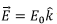which corresponds to a potential of φ = -E0r cos θ.

As before we can expand the potential inside and outside in terms of Legendre polynomials. Since the distant potential only has Legendre polynomial of order 1, we need to include only to include up to this order. Outside the sphere, the field is given by the potential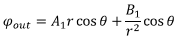Inside the sphere, the origin is included. Thus, inside the sphere, the potential cannot have a singularity at the origin and is given by

φin (r, θ) = A2r cos θ.
Comparing with the asymptotic limit at long distances A1 = -E0.

The potential itself is continuous at r=R,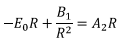Since there are no free charges on the surface, the normal component of the displacement vector is continuous across the surface. The normal component being the radial direction, we have,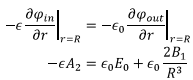Solving, we get,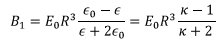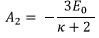so that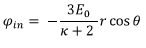and the electric field inside the dielectric is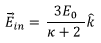It is seen that the due to the presence of dielectric the electric field inside is reduced by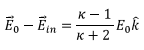We observe that if a dipole is kept at the origin of a sphere of radius R, since the radius of the sphere can be considered very large compared with the dimensions of the dipole, the field is given by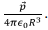Thus the effect of dielectric is the same as that of replacing the dielectric with a dipole of moment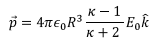The polarization of the sphere is then obtained by dividing this by the volume of the sphere, i.e., by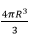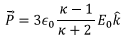Thus the field due to the dielectric can be written as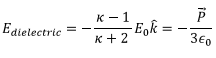Note that the polarization in the medium is uniform and is directed along the direction of the external field. The field lines approach the sphere from the left (negative z direction) and leave from the right, making the left face of the sphere negatively charged.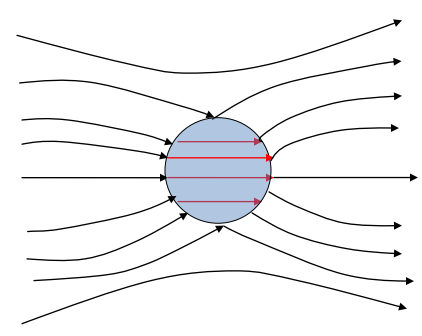Reduction in the electric field due to the presence of a dielectric

Consider a point charge in an isotropic dielectric with permittivity ∈. Taking the position of the charge at the origin, the field must be radial. The displacement field can be found from the Gauss’s law,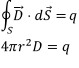so that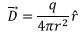Since the dielectric is uniform,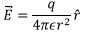Thus in the presence of a dielectric the electric field is reduced by a factor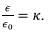The dielectric constant is a measure of the factor by which the electric field gets reduced in a linear dielectric medium. The polarization vector is given by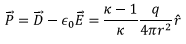Consider a Gaussian sphere of radius r. We can calculate the volume charge density inside the volume by the negative divergence of the polarization vector,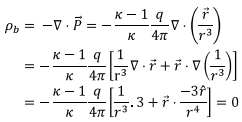However, there is a bound surface charge density. Since the dielectric is inside the sphere, the surface direction is inward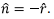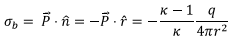Thus there exists a net negative bound charge. The total charge is obtained by multiplying the above with the surface area, viz., 4πr2 which gives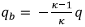as the total charge bound to the surface. The effective charge seen by a test charge is the sum of the original charge as reduced by this amount, i.e.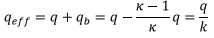i.e., the test charge experiences the electric field due to an effective charge screened by a factor equal to the dielectric constant of the medium.

Example : A parallel plate capacitor filled with a dielectric :

From the above discussion it is clear the electric field inside the dielectric will be reduced due to polarization effect of the dielectric. Consider a Gaussian pillbox in the shape of a rectangular parallelepiped of a very small thickness but of area a (A is the area of the plates)partly inside the medium through one of the plates and partly outside.

Since the plates have real charges, using Gauss’s law for the displacement vector, we have,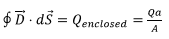Q here is the total free charge on the plate. Thus,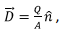the direction of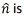from the negative plate towards the positive plate. The electric field in the dielectric is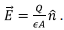The potential is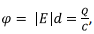which gives the capacitance to be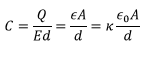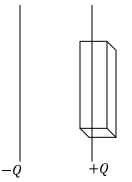Thus the capacitance is increased by a factor of k, the dielectric constant. This, of course, is true only for a linear dielectric.

Microscopic Theory

Before leaving the subject of electrostatics, we will briefly touch upon the molecular aspect of polarizability as opposed to a purely macroscopic treatment done so far.

We look at the dielectric as a collection of molecules. If the molecules are well separated, as would be the case in a gas, each molecule would experience an average macroscopic field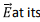site. This could consist of any external field and an average field due to all other molecules etc. The polarization induced is the written in terms of electric susceptibility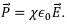For the case of a gas, the electric field is primarily due to the external field.

In a dense medium, however, this is not a good description because a particular molecule will be subject to a local field, which in addition to the external field, is due to interactions with the dipoles in its immediate neigbourhood as well as due to polarization of the other molecules in the medium. This local field induces a dipole moment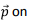the molecule in question and we define the “atomic polarizability” on the molecule in question and we define the “atomic polarizability” by the relation α by the relation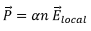If there are n atoms per unit volume, the polarization is given by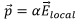However, since an atom cannot exert a force on itself, in computing the local field we must subtract the contribution to the polarization by the atom under consideration. For this purpose, let us divide the dielectric into two parts : a spherical volume of radius Rassigned to the atom in question which contains other atoms in the immediate neighborhood of the atom and the rest of the dielectric outside this volume. The “rest of the dielectric” will be treated in a macroscopic way which gives rise to an average field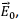taken along the z direction. To this field we must add the field which arise because of the spherical volume mentioned above.

The sphere can be treated as a cavity in an otherwise uniform medium. The inside surface of this cavity has a charge density σb corresponding to the uniform polarization.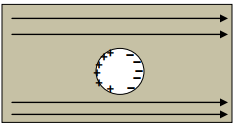Since the normal is directed inwards, the bound surface charge is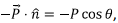where θ is the polar angle with respect to the z axis. The electric field due to this charge distribution is easily calculated,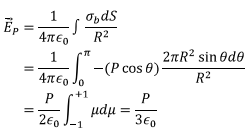Now we must look into the interaction of the dipoles within the cavity with the molecule (dipole) in question). We had seen earlier that the field due to a dipole at a position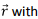respect to the dipole is given by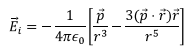The net field at the position of the atom is obtained by averaging the field due to all the dipoles. Since the molecules are oriented randomly, let us find the average of the field along the z direction,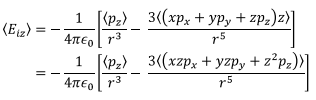Since the dielectric is isotropic, we have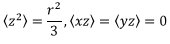We are left with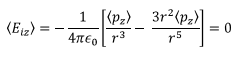Thus the total field is simply the sum of the first two contributions, i.e.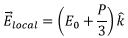Since the induced dipole moment is proportional to the local field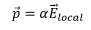So that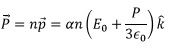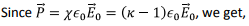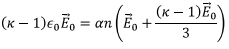so that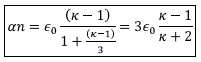This is called ClausiusMossotti Relation and it relates the atomic polarizability with the dielectric constant of the medium.

Energy of a charge distribution in a dielectric

In an earlier lecture we had obtained an expression for the energy of a charge distribution in free space. This was done by computing the work done in assembling the charges which are initially located at infinity. The expression for the energy was shown to be given by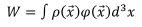In calculating the energy of a system of charges in a dielectric, we need to also take into account the work done in establishing the state of polarization of the dielectric.

Let us assume that at certain instant of time we have a charge density p already established and we add true charges to the system so that the charge density increases by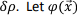be the potential in the presence of the charge density p. Since the charges are true charges, we have,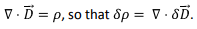The additional work that needs to be done to increase the charge density is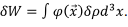Note that the factor of ½ that was there in the expression for the energy is not there because it had come to avoid double counting whereas in the present case we are just calculating the work done. Let us simplify the expression for the additional work done by integrating the above expression by parts,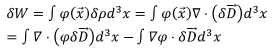The first term is converted to a surface integral using the divergence theorem. If we take the integration to be over all space, as has been pointed out on several occasion, the fields and potential vanish and this integral does not contribute to the energy. Using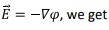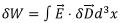The total work done in establishing the charge distribution is then obtained by integrating this expression from zero field situation to the final field configuration. For linear dielectrics, we have,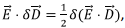, which can be used to get the energy expression as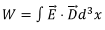Tutorial Assignment

1. A solid dielectric cylinder of dielectric constant k is placed in a uniform electric field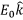perpendicular to its length. Obtain an expression for the potential inside and outside the cylinder.

2. A uniform electric fieldis set up in a medium of dielectric constant k. A spherical cavity of radius R is inside this medium. Find an expression for the electric field inside the cavity.

Solutions to Tutorial assignment

1. As the extent of the cylinder is infinite in the z direction, one can use polar coordinates to describe the potential. Since the far field pattern is φ(r) = - E0r cos θ, we take the following expressions to represent the potential (Note the difference between the solution in the polar case and that in the spherical case with azimuthal symmetry)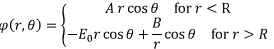On this we impose the boundary condition at the normal component of D and the tangential component of E field are continuous.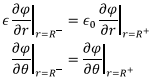Use these to express A and B in terms of E0. The result is2. Assume the dielectric to fill the entire space with the cavity centred at r=0. The problem has aqzimuthal symmetry. The potential is given by the expression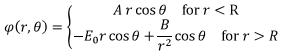On this we impose the boundary condition at the normal component of D and the tangential component of E field are continuous.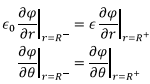These give (as the relations are valid for all values of θ)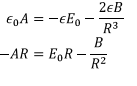Solving, we get,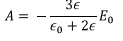Thus the potential is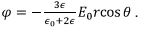The corresponding electric field is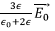Self Assessment Quiz
1. A parallel plate capacitor is filled with a linear dielectric whose dielectric constant varies linearly from the grounded bottom plate to the upper plate located at a distance d, by the relation k = k0 +cz. If the upper plate is at a potential of φ0, obtain expressions for (a) the potential as a function of z, (b) the electric field in the dielectric and (b) the polarization charge densities on the plates.

2. The space between the plates of a parallel plate capacitor is filled with two slabs of dielectric material. The dielectric filling the space between the grounded bottom plate at z=0 up to z=d/2 is of dielectric constant k1 while that filling the space from z=d/2 up to the upper plate at z=d which is maintained at a constant potential φis of dielectric constant k2. Find an expression for the potential between the plates and the polarization charge density on the two plates.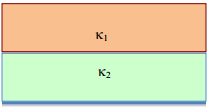Solutions to Self Assessment Quiz

1. We start with an expression for the D field in the dielectric. As the plates contain free charge densities, the D field has a strength Q/A directed from the positive plate to the negative plate. The electric field is then given by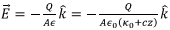One can calculate the potential at any point by evaluating the line integral of the electric field from the bottom plate to a point at a distance z,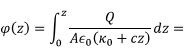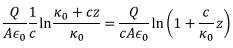Substituting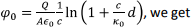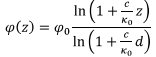The expression for the electric field can be obtained by taking the negative gradient of the potential or by expressing the previously obtained expression in terms of given constants,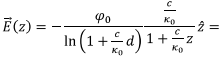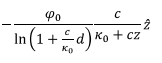Thus the displacement vector is given by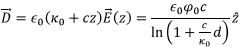Which is of course constant. The polarization vector is given by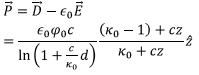The polarization charge densities on the plates is given by the normal component of the polarization vector. On the upper plate, z=d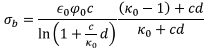On the lower plate z=0,2. Let the charge on the plates be +Q and the area of the plates be A. The displacement vector is D=Q/A. The electric field from z=0 to z= d/2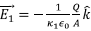and that in the region from z=d/2 to z=d is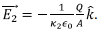The potential in the region 1 is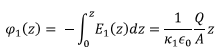The potential at z=d/2 is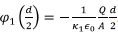. The potential in the region 2 is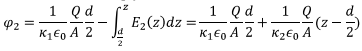The potential at z=d is then given by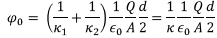where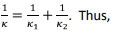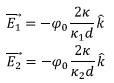The charge density is given by the normal component of the polarization vector,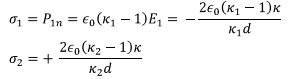Offer running on EduRev: Apply code STAYHOME200 to get INR 200 off on our premium plan EduRev Infinity!

,

,

,

,

,

,

,

,

,

,

,

,

,

,

,

,

,

,

,

,

,

;